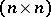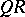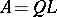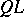# Triangular matrix

Any-matrixof rankin which the firstsuccessive principal minors are different from zero can be written as a product of a lower triangular matrixand an upper triangular matrix, [a1].
Any real matrixcan be decomposed in the form, whereis orthogonal andis upper triangular, a so-called-decomposition, or in the form, withorthogonal andlower triangular, a-decomposition or-factorization. Such decompositions play an important role in numerical algorithms, [a2], [a3] (for instance, in computing eigenvalues).
Ifis non-singular andis required to have its diagonal elements positive, then the-decompositionis unique, [a3], and is given by the Gram–Schmidt orthonormalization procedure, cf. Orthogonalization; Iwasawa decomposition.